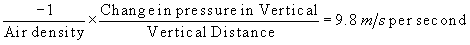### Meteorology: Understanding the Atmosphere            Ackerman and Knox

.

How can we adjust Denver’s pressure to sea level? A city at sea level, such as San Francisco, has one more mile of air above it than Denver. To adjust the Denver pressure for sea level we have to add the pressure caused by the difference resulting from that extra mile of air. (What's the math?)

Use the applet to consider the following questions; Set the measured pressure to 900 mb and the station altitude to 500 m. How sensitive is the correction to sea level with respect to temperature? Set the average temperature to 10 C (50 F) and the station altitude to 500 m. How sensitive is the correction to sea level with respect to measured pressure?

## The mathematics

The ideal gas law can also be written as

Pressure = R x Density x Temperature

where R is a constant that depends on the gases that compose the atmosphere. For dry air, the value of R is 287.05 Joules per kilogram per degree Kelvin.

Remember that Density is mass per unit volume. In Chapter 6 we discuss Hydrostatice balance and introduce the equation as:Combining these two equations yields what is called the hypsometric equation:orwhere Z1 is sea level (or zero), Z2 is the station altitude, P2 is the measured pressure, T is a representative temperature. From these, the corrected pressure, P1, is calculated.

This applet computes an estimated sea level pressure given the measured pressure, the altitude of the weather station, and a representative mean temperature between the station and sea level (C). You must hit the return key after entering each number. As Box 1.1 explains, moist air is lighter than dry air so we should include moisture in this calculations - a topic to be addressed as part of Chapter 4.